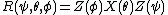# Euler Angles

(redirected from Euler angle)

## Euler angles

Three angular parameters that specify the orientation of a body with respect to reference axes. They are used for describing rotating systems such as gyroscopes, tops, molecules, and nonspherical nuclei. They are not symmetrical in the three angles but are simpler to use than other rotational parameters.

Unfortunately, different definitions of Euler's angles are used, and therefore it is confusing to compare equations in different references. The definition given here is the majority convention according to H. Margenau and G. Murphy.

Let OXYZ be a right-handed cartesian (right-angled) set of fixed coordinate axes and Oxyz a set attached to the rotating body (see illustration).

The orientation of Oxyz can be produced by three successive rotations about the fixed axes starting with Oxyz parallel to OXYZ. Rotate through (1) the angle ψ counterclockwise about OZ, (2) the angle Θ counterclockwise about OX, and (3) the angle &phgr; counterclockwise about OZ again. The line of intersection OK of the xy and XY planes is called the line of nodes.

Denote a rotation about OZ, for example, by Z (angle). Then the complete rotation is, symbolically, given by the equation below where the rightmost operation is donefirst.

McGraw-Hill Concise Encyclopedia of Physics. © 2002 by The McGraw-Hill Companies, Inc.
The following article is from The Great Soviet Encyclopedia (1979). It might be outdated or ideologically biased.

## Euler Angles

(or Eulerian angles), the angles φ, θ, and ψ that define the position of a rectangular Cartesian coordinate system OXYZ with respect to another rectangular Cartesian coordinate system Oxyz of the same orientation (see Figure 1).Figure 1

Let OK be an axis, called the line of nodes, that coincides with the line of intersection of the coordinate plane Oxy of the first system with the coordinate plane OXY of the second system and is directed so that the axes Oz, OZ, and OK form a trihedral of the same orientation. The Euler angle φ, or angle of spin, is the angle between the axes Ox and OK, which is measured in the plane Oxy from Ox in the direction of the shortest rotation from Ox to Oy. The Euler angle θ, or angle of nutation, is the angle between Oz and OZ; it does not exceed π. The Euler angle ψ, or angle of precession, is the angle between the axes OK and OX, which is measured in the plane OXY from Ok in the direction of the shortest rotation from OX to OY. When θ = 0 or π, the Euler angles are not defined.

The Euler angles were introduced by L. Euler in 1748 and are used extensively in the dynamics of solids—for example, in the theory of the gyroscope—and in celestial mechanics.

## Euler angles

[′ȯi·lər ‚aŋ·gəlz]
(mechanics)
Three angular parameters that specify the orientation of a body with respect to reference axes.
McGraw-Hill Dictionary of Scientific & Technical Terms, 6E, Copyright © 2003 by The McGraw-Hill Companies, Inc.
References in periodicals archive ?
where d[phi], d[theta], and d[psi] are the roll, pitch, and heading difference in Euler angle between INS outputs and smoothed estimates.
In Euler's theorem, an attitude can be represented by a single rotation angle about a principal axis, called the eigen-axis, or three sequential rotation angles, called the Euler angles. A rest-to-rest maneuver to a target attitude can then be performed by either an eigen-axis maneuver or three sequential principal submaneuvers following Euler angle sequences.
Therefore it is important to provide a basis to the antenna pattern angle definitions and aeronautical platform Euler angle definitions as in Figures 2 and 3, respectively.
There are three main approaches for attitude representation: DCM, Euler angle, and quaternion.
The variables input to each channel were angular velocity, Euler angle, Euler angular error, Euler angular error differential value, and each control surface deflection angle.
The variation in the phase velocity ([V.sub.p]) and coupling factor ([K.sup.2]) of SAWs with the top electrode thickness, thickness, and Euler angle (0) of the YX-PIMNT substrate was investigated.
The Euler angle parameterization is not global, and angles are not well-defined for [theta] = [+ or -][pi]/2.
A MEMS IMU and a two-antenna GPS are fused to determine the vehicle attitude for vehicle-mounted satellite-communication Satcom-on-the-move (SOTM), using an adaptive Euler angle based unscented Kalman filter (UKF) .
In this article, based on the polarization properties of Raman spectra, the step-by-step measuring method for the Euler angle of the wurtzitic crystal is proposed.
The most common algorithm of the projection matching refinement methods starts from a coarse initial angular increment (the second Euler angle [phi]), obtaining quasi-even distributions of projection directions on the unit sphere [S.sup.2].
The motion of a projectile in atmospheric flight is assumed to be adequately described with six rigid body degrees of freedom comprised of three inertial position body coordinates as well as three Euler angle body attitudes.

Site: Follow: Share:
Open / Close